Basics of Geometry
edHelper subscribers - Create a new printable

Number of Keys
 Select the number of different printables: 1 key 2 keys 3 keys 4 keys 5 keys

Number of Pages (for each key)
 Select the number of pages: 1 page 2 pages 3 pages 4 pages

Include an answer key (answer keys will be at the end of the printable)
High School Geometry
High School Geometry
Math

 Name _____________________________Date ___________________ (Key 1 - Answer ID # 0474694)
1.   If the number of sides in a polygon was doubled, the sum of its interior angles would increase by 1,260. How many sides does the original polygon have?
2.   What is the number of sides in a regular polygon in which the measure of an interior angle is twelve more than six times the measure of an exterior angle?
3.   What is the measure of each interior angle in a regular hexagon?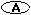144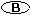120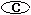210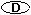90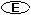120
4.   How many diagonals can be drawn inside of a heptagon?141713None of the above
5.   Polygon P has t sides. How many diagonals can be drawn inside of polygon P?4 (t - 2)t(t - 3) ÷ 22 (t)t(t - 2) ÷ 3t(t - 2) ÷ 2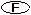t(t - 2)
6.   If the sum of the measures of polygon is 2160, how many sides does the polygon have?241711202114
7.   Each point of a polygon at which two sides intersect is called ___________.a sidea vertexexterior angleinterior anglediagonal
8.   Polygon C has h sides. What is the sum of the measures of polygon's C interior angles?h(h - 3) ÷ 2180 (h - 2) ÷ 2360 (h - 2)90 (h - 4)180 (h - 2)

Basics of Geometry
edHelper subscribers - Create a new printable

Number of Keys
 Select the number of different printables: 1 key 2 keys 3 keys 4 keys 5 keys

Number of Pages (for each key)
 Select the number of pages: 1 page 2 pages 3 pages 4 pages